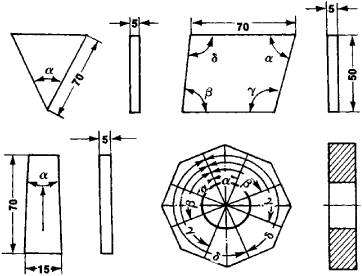# Angular Measures

## Measures, Angular

measures that are used to reproduce angles of given value. Angular measures can be single-valued or multivalued. Angle blocks are single-valued angular measures; some of the multivalued angular measures are polyhedral prisms (see Figure 1), limbs, and circular scales. Angle blocks are steel blocks 5 mm thick with one or four dihedral angles formed by the lateral faces of the block. Blocks with working angles of one minute (1’) to 100° are assembled into sets consisting of 93, 33, or fewer measures in such a way that from three to five measures can be combined into blocks having intervals of 1°, 1′, or 15 seconds (15″). Special holders are used to assemble angle blocks into sets. Angle blocks are made in three classes of precision: class 0, class 1, and class 2, with errors up to 3” for class 0 and up to 30″ for class 2.Figure 1. Prismatic angular measures (Greek letters denote the angles reproduced by the measures, and dimensions are given in millimeters)

Polyhedral prisms are made of glass, fused quartz, and steel; the number of faces is usually as high as 36 and is sometimes as high as 72. Permissible deviations of working angles are from ± 5′ for class 0 to ± 30″; for class 2.

Limbs are usually a part of various angle-measuring instruments, such as goniometers, theodolites, quadrants, and dividing heads. Limbs are made with various accuracies, with scale divisions from 1′to 10′ and above, and with errors from 1″ to 10″.

According to their metrological purpose, angular measures are classified as base standards and working standards.

### REFERENCES

GOST 2875–62: Mery uglovye prismaticheskie.
Eidinov, V. la. Izmerenie uglov v mashinostroenii. Moscow, 1963.

K. P. SHIROKOV

References in periodicals archive ?
Volume 1, like Burnham's, begins with a roughly 100-page introduction to the astronomy that an amateur really needs to know: the celestial sphere and the sky's motions, the magnitude system, angular measures, and so on, along with lots about the various types of objects to be seen in amateur scopes.
The following linear and angular measures were applied:
When applied as indicated, is an excellent method to assess both linear and angular measures on images where the planification of structures doesn't alter the real 3D image dimensions.
The 15 dimensional or angular measures in Groups 1 through 3 and the three cone volume measures were treated differently in the regression analyses based on the same model.
To illustrate subtraction at the manipulative level, for example, the authors suggest activities that involve base-ten blocks, body measurements, angular measures, grocery problems, dice, shading rectangles, and number games.

Site: Follow: Share:
Open / Close